## A committee of 3 is to be formed from 5 men and 6 women. (a) What is the probability that a random committee is made up of 2 women and 1 man

Question

A committee of 3 is to be formed from 5 men and 6 women. (a) What is the probability that a random committee is made up of 2 women and 1 man? (b) Suppose now that the committee consists of different positions: Chairperson, Vice-Chairperson, and Treasurer. What is the probability that the Chairperson and Vice Chairperson are both women and the Treasurer is a man?

in progress 0
2 months 2021-07-21T16:57:53+00:00 1 Answers 1 views 0

a) 0.4545 = 45.45% probability that a random committee is made up of 2 women and 1 man.

b) 0.1515 = 15.15% probability that the Chairperson and Vice Chairperson are both women and the Treasurer is a man

Step-by-step explanation:

A probability is the number of desired outcomes divided by the number of total outcomes.

If the order of the people chosen is important, we use the permutations formula. If the order is not important, we use the combinations formula.

Permutations formula:

The number of possible permutations of x elements from a set of n elements is given by the following formula: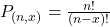Combinations formula: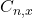is the number of different combinations of x objects from a set of n elements, given by the following formula.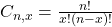(a) What is the probability that a random committee is made up of 2 women and 1 man?

Order is not important, so we use the combinations formula.

Desired outcomes:

2 women from a set of 6, and a men from a set of 5. So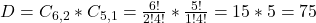Total outcomes:

3 people from a set of 6 + 5 = 11. So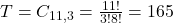Probability: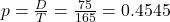0.4545 = 45.45% probability that a random committee is made up of 2 women and 1 man.

(b) Suppose now that the committee consists of different positions: Chairperson, Vice-Chairperson, and Treasurer. What is the probability that the Chairperson and Vice Chairperson are both women and the Treasurer is a man?

Now, the order is important, which means that we use the permutations formula.

Desired outcomes:

2 women from a set of 6, and a men from a set of 5. So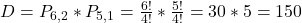Total outcomes:

3 people from a set of 11. So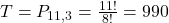Probability: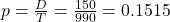0.1515 = 15.15% probability that the Chairperson and Vice Chairperson are both women and the Treasurer is a man